# DAV Class 8 Maths Chapter 12 Worksheet 3 Solutions

The DAV Maths Class 8 Solutions and DAV Class 8 Maths Chapter 12 Worksheet 3 Solutions of Construction of Quadrilaterals offer comprehensive answers to textbook questions.

## DAV Class 8 Maths Ch 12 WS 3 Solutions

Question 1.
Using ruler and compass only:
(i) Construct a quadrilateral ABCD in which $$\overline{\mathrm{AB}}$$ = 3.5 cm, $$\overline{\mathrm{BC}}$$ = 6.5 cm, ∠A = 60°, ∠C = 120° and ∠D = 75°.
Solution:
Steps of Construction:
1. Draw $$\overline{\mathrm{AB}}$$ = 3.5 cm.
2. Draw an angle of 105° [∵ 360° – (75° + 120° + 60°)] at B and cut $$\overline{\mathrm{BC}}$$ = 6.5 cm.
3. Draw an angle of 120° at C and 60° at A to meet at D.
4. Join $$\overline{\mathrm{CD}}$$ and $$\overline{\mathrm{AD}}$$.
5. ABCD is the required quadrilateral.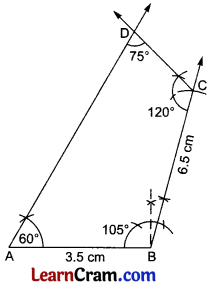(ii) Construct a quadrilateral ABCD in which $$\overline{\mathrm{AB}}$$ = 4 cm, $$\overline{\mathrm{BC}}$$ = 7 cm, ∠A = ∠C = 105° and ∠D = 60°.
Solution:
Steps of Construction:
1. Draw $$\overline{\mathrm{AB}}$$ = 4 cm.
2. Draw an angle of 90° at B. [∵ 360° – (105° + 105° + 60°) = 90°]
3. Cut $$\overline{\mathrm{BC}}$$ = 7 cm.
4. Draw angles of 105° at C and at A each to meet at D.
5. ∠D will come to 60°.
6. ABCD is the required quadrilateral.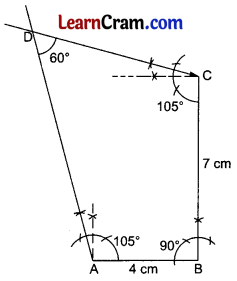(iii) Construct a quadrilateral PQRS in which $$\overline{\mathrm{PQ}}$$ = 5 cm, $$\overline{\mathrm{QR}}$$ = 6 cm, ∠P = 75°, ∠Q = 105° and ∠R = 90°.
Solution:
Steps of Construction:
1. Draw $$\overline{\mathrm{PQ}}$$ = 5 cm.
2. Draw an angle of 105° at Q and cut $$\overline{\mathrm{QR}}$$ = 6 cm.
3. Draw an angle of 90° at R and 75° at P to meet at S.
4. PQRS is the required quadrilateral.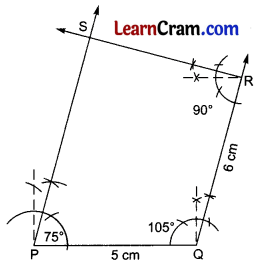(iv) Construct a quadrilateral ABCD in which $$\overline{\mathrm{AB}}$$ = 4.5 cm, $$\overline{\mathrm{BC}}$$ = 5 cm, ∠A = 60°, ∠B = 120° and ∠C = 60°.
Solution:
Steps of Construction:
1. Draw $$\overline{\mathrm{AB}}$$ = 4.5 cm.
2. Draw an angle of 120° at B and cut $$\overline{\mathrm{BC}}$$ = 5 cm.
3. Diaw an angle of 60° at C and A each to meet at D.
4. ABCD is the required quadrilateral.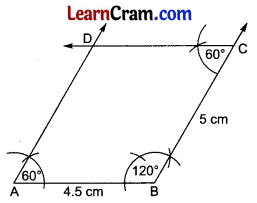Question 2.
If necessary, construct the following quadrilaterals using a ruler, compasses, and protractors.
(i) A quadrilateral ABCD in which $$\overline{\mathrm{AB}}$$ = 4 cm, $$\overline{\mathrm{BC}}$$ = 4 cm, ∠A = 70°, ∠B = 80° and ∠C = 90°.
Solution:
Steps of Construction:
1. Draw $$\overline{\mathrm{AB}}$$ = 4 cm.
2. Draw an angle of 80° with protractor at B and cut BC = 4 cm.
3. Draw an angle of 90° at C and 70° at D to meet at D.
4. ABCD is the required quadrilateral.(ii) A quadrilateral PQRS in which $$\overline{\mathrm{PQ}}$$ = $$\overline{\mathrm{QR}}$$ = 5 cm, ∠P = 100°, ∠Q = 80° and ∠R = 100°.
Solution:
Steps of Construction:
1. Draw $$\overline{\mathrm{PQ}}$$ = 5 cm.
2. Draw an angle of 80° at Q and cut $$\overline{\mathrm{QR}}$$ = 5 cm.
3. Draw an angle of 100° at R and at P both to meet at S.
4. PQRS is the required quadrilateral.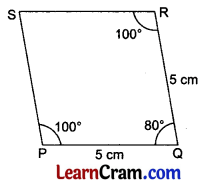(iii) A quadrilateral ABCD in which $$\overline{\mathrm{AB}}$$ = 5 cm, $$\overline{\mathrm{BC}}$$ = 4 cm, ∠A = 80°, ∠B = 90° and ∠C = 100°.
Solution:
Steps of Construction:
1. Draw $$\overline{\mathrm{AB}}$$ = 5 cm.
2. Draw an angle of 90° at B and cut $$\overline{\mathrm{BC}}$$ = 4 cm.
3. Draw an angle of 100° at C and 80° at A to meet at D.
4. ABCD is the required quadrilateral.